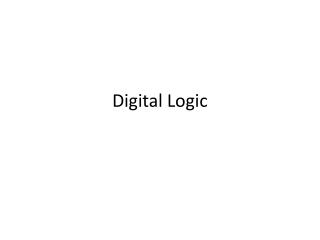# Digital Logic - PowerPoint PPT PresentationDownload PresentationDigital Logic

Digital Logic
Download Presentation## Digital Logic

- - - - - - - - - - - - - - - - - - - - - - - - - - - E N D - - - - - - - - - - - - - - - - - - - - - - - - - - -
##### Presentation Transcript

1. Digital Logic

2. I O GND I O Resister (limits conductivity) Vcc Abstractions in CS (gates) • Basic Gate: Inverter Truth Table I O 0 1 1 0

3. I O Abstractions in CS (gates) Truth Table I O 0 1 1 0

4. A B Y A B Y 0 0 1 1 0 1 0 1 1 1 1 0 GND A B Y Vcc Abstractions in CS (gates) • Basic Gate: NAND (Negated AND) Truth Table

5. A B Y 0 0 1 1 0 1 0 1 0 0 0 1 Abstractions in CS (gates) • Basic Gate: AND Truth Table A B Y

6. A B Y 0 0 1 1 0 1 0 1 0 1 1 1 Abstractions in CS (gates) • Other Basic Gates: OR gate Truth Table A B Y

7. A B Y 0 0 1 1 0 1 0 1 0 1 1 0 Abstractions in CS (gates) • Other Basic Gates: XOR gate Truth Table A B Y

8. Logic Blocks • Logic blocks are built from gates that implement basic logic functions • Any logical function can be constructed using AND gates, OR gates, and inversion.

9. Adder • In computers, the most common task is add. • In MIPS, we write “add \$t0, \$t1, \$t2.” The hardware will get the values of \$t1 and \$t2, feed them to an adder, and store the result back to \$t0. • So how the adder is implemented?

10. Half-adder • How to implement a half-adder with logic gates? • A half adder takes two inputs, a and b, and generates two outputs, sum and carry. The inputs and outputs are all one-bit values. a sum b carry

11. Half-adder • First, how many possible combinations of inputs?

13. Half-adder • The value of sum should be? Carry?

14. Half-adder • Okay. We have two outputs. But let’s implement them one by one. • First, how to get sum? Hint: look at the truth table.

15. Half-adder • Sum a sum b

16. How about carry? • The truth table is

17. Carry • So, the circuit for carry is a carry b

18. Half-adder • Put them together, we get a sum b carry

20. Constructing Truth Table for 1-Bit Adder

21. Truth Table for a 1-Bit Adder

22. Sum? • Sum is `1’ when one of the following four cases is true: • a=1, b=0, c=0 • a=0, b=1, c=0 • a=0, b=0, c=1 • a=1, b=1, c=1

23. Sum • The idea is that we will build a circuit made of and gates and or gate faithfully according to the truth table. • Each and gate corresponds to one ``true’’ row in the truth table. The and gate should output a ```1’’ if and only if the input combination is the same as this row. If all other cases, the output is ``0.’’ • So, whenever the input combination falls in one of the ``true’’ rows, one of the and gates is ``1’’, so the output of the or gate is 1. • If the input combination does not fall into any of the ``true’’ rows, none of the and gates will output a ``1’’, so the output of the or gate is 0.

24. Boolean Algebra • We express logic functions using logic equations using Boolean algebra • The OR operator is written as +, as in A + B. • The AND operator is written as ·, as A · B. • The unary operator NOT is written as . or A’. • Remember: This is not the binary field. Here 0+0=0, 0+1=1+0=1, 1+1=1.

25. Sum

26. Carryout bit? • Carryout bit is ‘1’ also on four cases. When a, b and carryin are 110, 101, 011, 111. • Does it mean that we need a similar circuit as sum?

27. Carryout bit • Actually, it can be simpler

28. Delay • Hardware has delays. • Delay is defined as the time since the input is stable to the time when the output is stable. • How much delay do you think the one-bit half adder is and the one-bit full adder is?